# 5 – Tabular Amasser

 < ρℝεν | ℂσητεητs | ℕεχτ >“It is a curious historical fact that modern quantum mechanics began with two quite different mathematical formulations: the differential equation of Schroedinger and the matrix algebra of Heisenberg. The two apparently dissimilar approaches were proved to be mathematically equivalent.” – Richard P. Feynman

The Matrix is Everywhere

This chapter should probably start with an apology for the rather awful pun contained in its title. However my mangling of an element of John Locke’s An Essay Concerning Human Understanding perhaps masks a seed of truth. Here I will look to describe the Mathematical construct known as matrices. A matrix is something that should be familiar enough to any user of Excel or Word, it’s just a table. Instead of the contents being last month’s sales figures by division or some random text, these Mathematical tables include – perhaps unsurprisingly – just numbers (or algebraic symbols, like “a”, standing for numbers).

A typical matrix might look something like this (where the entries are Integers):This is a 3 × 4 matrix, one with three rows and four columns. A number is entered at each intersection of columns and rows. Two dimensional matrices in general have n columns and m rows, with a number recorded in each place in the table. Most of the matrices I deal with in this Chapter will be square (n columns and n rows) for reasons we’ll cover in a bit, however there is in general no such restriction placed on their sizes .

You can think of matrices as being an extension to the concept of number. Instead of thinking about single numbers, we can think of a group (small “g”) of these collectively. To look at it another way, 1 × 1 matrices are essentially just the numbers we normally deal with.

An obvious question is why do we need tables of numbers? Aside from the fact that you can do some interesting Mathematics with them, matrices actually have many real world applications, particularly where several facts are to be recorded simultaneously.

The following example is somewhat artificial and also quite different to how matrices are actually used in various branches of Science. However, I think that it is relatively easy to understand and provides at least a flavour of what matrices do. For these two reasons I’m going to stick with this somewhat flawed example and beg the forgiveness of any more numerically astute readers in advance. If you are quite happy to accept that matrices have many real world applications, then please feel free to skip the box.

 What have Matrices ever done for us? Let’s think about a particle moving in a flat two dimensional space. By two dimensional space I mean a two dimensional surface (like the top of a table, or a sheet of paper – but extending to infinity in both directions) together with axes (lines with gradations marked on them, like a ruler) which help us to anchor where things are in the space. This ends up looking a lot like the type of graph paper school children use to plot graphs on. See below:Different to most rulers, our axes extend both downwards and leftwards beyond zero to include negative lengths (lengths in the opposite direction to the one we are measuring), where they cross at length zero in both directions is called the origin. Traditionally the vertical axis is labelled y and the horizontal one x. We now have a frame of reference to consider our particle. In Classical (Newtonian) Mechanics, its current state is defined by both where it is and how it is moving. Looking at the diagram above, if our particle is the red dot, then we can see that it is located 18 units from the origin on the x axis and 13 units from the origin on the y axis. How is it moving? The red arrow shows its current direction of travel and its length shows its speed (in units per second). It is very typical to decompose such movement in to the element in the direction of each of the two axes; this helps with calculations. Taking this approach, the two black arrows indicate that it is moving at 9 units per second along the x axis and 5 units per second up the y axis . The point I am looking to convey here is that the current state of our particle can be wholly characterised by just four numbers: 18, 13, 9, 5. Further these numbers are of two kinds. Two are locations in space and two are speeds. We might want to record these numbers in a table as follows:More generally the current state of our particle at any point could be captured by: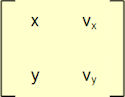How the values of x, y, vx and vy change over time depends on many things. If the particle is experiencing no other force, it will continue in a straight line and so its position t seconds later will be given by: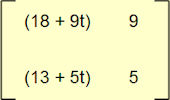Here the x and y values change, but its speed is constant . However if it is acted on by some force, it will accelerate and in this case both its location and the components of its speed will vary . This is not a book about Mechanics, so I’m not going to work through what the entries might look like relative to different accelerations. The point is this: we can record multiple pieces of information about a particle in a table and also show how the state of this particle changes over time using the same approach. In some sense the overall table embodies the particle. This is the essence of how matrices are applied to physical situations, though the details may vary greatly from my rather simplistic example .

Laying our Cards on the TableSo far I have spoken about a matrix as being a way to collect together different numbers and we have looked at equations operating on these numbers in some way, with the results also being captured in a matrix (we even had one matrix that included such equations; things like 18 + 9t, where t is time). What about performing operations (maybe even binary operations) on matrices themselves?

Before getting into this, a comment on notation. While to date I have spelled out the tables and rows of matrices, it is typical to refer to the whole matrix by a capital letter, for example: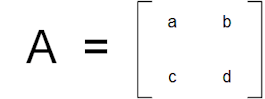I’m going to look at our two familiar binary operations addition and multiplication. As before we need to be precise in what we mean by these. Addition is the simpler of the two. For two matrices, say A and B, A + B is achieved by adding the individual matrix elements together as in: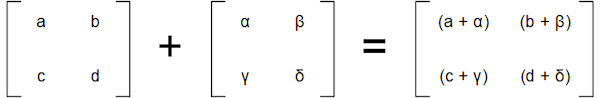It should be noted that both matrices have to be of the same size (same number of rows and same number of columns) for this to work.

For multiplication, there are two concepts to consider, scalar multiplication and matrix multiplication. Scalar  multiplication relates to multiplying a matrix by a number, so 3A or more generically λA. Scalar multiplication involves multiplying all elements of a matrix, A, by this number, as per:What we are more interested in here is matrix multiplication, i.e. multiplying two matrices A and B. We might be tempted to define A × B in the same sort of way as addition, i.e.:However, this definition has no real mathematical properties beyond those inherent in each of the individual multiplications. There is also no real sense of the two matrices being multiplied, only their contents. Instead matrix multiplication is generally defined as a more complex operation, which has a strong relation to the concept of the dot product of vectors . This is a bit fiddly, so please bear with me as I explain what we do.

The rule for normal matrix multiplication, A × B, is as follows.

1. Take each cell of the output matrix (the one on the right of the equation) in turn and work out which row and column the cell sits in. Let’s call these row X and column Y.

2. Next look at the input matrices (the ones on the left of the equals sign). In the first input matrix, take each value from the row which is defined by the location of the output cell, so row X in this example.

3. Multiply each one of these values in turn by the figures in the second input matrix taken from the column which is also defined by the location of the output cell, so column Y in this example.

4. Finally add these together and place the result in row X and column Y of the output matrix.

It’s probably much easier to show this than to write it. So let’s see what happens if we look at the simplest two matrices we can multiply (apart from 1 × 1 matrices, which as we have mentioned are basically just regular numbers), then the process we just described plays out like this:Here note that a 1 × 2 matrix multiplied by a 2 × 1 matrix yields a 1 × 1 matrix. It is also worth observing that our rule does not allow us to multiply the same two matrices the other way round. Not least to avoid such complications, we will be mostly considering square matrices from now on.

If we now we go back to the two generic 2 × 2 matrices we were looking at before, then this is what happens:If we look at the upper left-hand entry in our results matrix (numbers highlighted in blue) we take the upper row of the first matrix to be multiplied (shown in a blue dashed box) and the left-hand column of the second matrix to be multiplied (again in a blue dashed box) and multiply each pair of numbers, adding the result together. The process is also shown, this time in red, for the bottom right-hand cell of the output matrix.

This definition of multiplication can be extended to bigger matrices. As in our first example of a 1 × 2 matrix multiplied by a 2 × 1 matrix, these do not have to be square. Matrix multiplication works so long as the number of columns in the first matrix equals the number of rows in the second. If we multiply a p × q matrix by a q × r matrix (noting that q must be the same in both cases) then the result is a p × r matrix .

We can employ our newly defined operation of matrix multiplication to look at our example of a particle’s position after t seconds in a different way: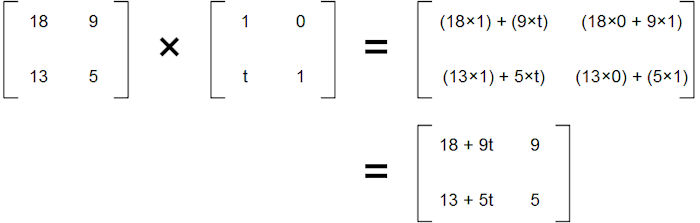Here the trick is performed by the introduction of the term t in the bottom left-hand cell of the second matrix. We’ll see more of this sort of thing later.

The Perennial Question

If you have read this far, then I am sure you will anticipate the question that is coming next; do matrices form Groups? The perhaps unsurprising answer is yes. Let’s focus on the set of 2 × 2 matrices with entries all in ℝ, the Real Numbers that we met in the last Chapter. We can write this as M2(ℝ) and then consider the binary operator of matrix multiplication using the definition we have just provided . We should by now be familiar with the list of things that we need to check:

1. Closure

By our previous observations – and looking at the generic definition of matrix multiplication above, it is evident that multiplying a 2 × 2 matrix by another 2 × 2 matrix yields a 2 × 2 matrix. If all of the entries are in ℝ, then this is closed under regular multiplication and regular addition, so all the entries in the resulting matrix will also be in ℝ.

2. Identity

Here we need to exercise some caution. The obvious candidate that springs to mind might well be the following matrix:But let’s work through what happens if we apply this to a generic 2 × 2 matrix:So this is a less fruitful approach than might have been anticipated. The matrix we used above to introduce a time factor to the observations about a particle actually points to the right solution, a matrix as follows:Let’s do the same test that the previous identity candidate failed and see how this new matrix fares:So this seems to work pretty well.

3. Inverses

If we have a 2 × 2 matrix and label this as A, then what we are looking for is another 2 × 2 matrix A-1 which when multiplied by A gives us the identity matrix:

AA-1 = e

remembering that e is our general label for any identity element, though many people (including me at points in this book) will use either 1 or I to also mean the n × n identity matrix, things that are fairly natural to do.

We can leverage our previous example of multiplying two generic 2 × 2 matrices together (the one highlighted in blue and red which appears above) to investigate this further. Let’s call the first matrix A; it has values {a, b} {c, d}. The second matrix has values {α, β}{γ, δ}. If we want this second matrix to be A’s inverse, or A-1, then we need to work out what values {α, β}{γ, δ} need to take. Using the definition of matrix multiplication, {α, β}{γ, δ} must satisfy the following equation:Let’s think about the top right and bottom left cells. We have:

aβ + bδ = 0

Which means that one possible solution is:

β = -b and δ = a, which would give us -ab + ba = 0

and also:

cα + dγ = 0

Which means that one possible solution is:

α = d and γ = -c, which would give us cd – dc = 0

Let’s try our possible solutions as a candidate for an inverse and see how far this gets us: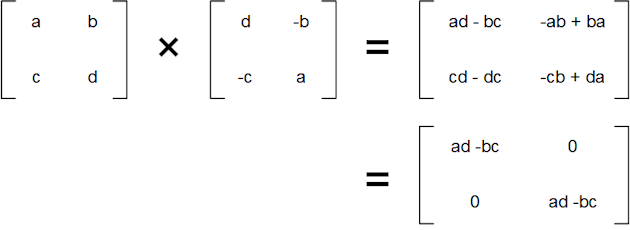Well that worked out pretty well, but instead of having 1s on the diagonal, we have numbers different to 1, specifically ad – bc in both cases. This number has a name, it is called the determinant of a matrix and we will come across it again later. For now, we need to complete our definition of an inverse by dividing the whole candidate matrix by these numbers to get: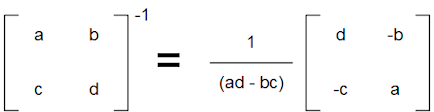So we have inverses.

However, there is a further point to be made here. What of a matrix where it happens that bc = ad, i.e. the determinant is zero? Generally dividing by zero in Mathematics is taboo. Therefore we are going to have to accept a restriction on our set of 2 × 2 (and indeed n × n) matrices. If we are looking for a Group, we need to consider only those matrices A, where the determinant of A, written det(A), is non-zero.

4. Associativity

As this property tends to generally be the last one tested and also as testing it properly tends to often be fiddly, you will frequently see some hand waving and “of course it may be seen” at this point.

Without doing a long-hand proof involving three matrices, it perhaps suffices to say that any of the entries in the final matrix will be the result of multiplications and additions and nothing else. As both of these are associative, so is multiplication of 2 × 2 matrices. Of course please feel free to do the proof more rigorously if you feel like it; I guarantee you will come to the same conclusion.

So once more we have discovered yet another Group. Because we had to ignore matrices whose determinant is zero, this Group is not M2(ℝ); instead it is a subset of this consisting of all invertible 2 × 2 matrices . This subset is called GL2(ℝ) for the General Linear Group of degree 2 over the Real Numvers. This is also a further example of a non-Abelian Group; when multiplying two matrices A and B, A × B is not the same as B × A. Indeed – as we have seen above – if we generalise to non-square matrices, multiplication may be defined for A × B but not for B × A.

It may be shown that this result for 2 × 2 matrices can be extended for any square matrix. The Group of n × n invertible matrices over ℝ is written as GLn(ℝ), which is a subset Mn(ℝ), i.e. all n × n matrices over ℝ.

It would be feasible to write a whole book of the same size as this one just on the subject of matrices, their properties and their application to many fields of human endeavour. Indeed it would be not that hard to pen a multi-volume set of books. We will be coming back to this area in Chapter 13, but before we do so there are a few aspects of matrices that I’d like to cover in some more detail. These are their properties relating to reflection and rotation and this is the subject of the next Chapter.

 Concepts Introduced in this Chapter Matrix A rectangular table of numbers with n rows and m columns. Matrices have many applications in Mathematics, Science and Engineering. Matrix Addition For two identically shaped matrices, adding the values appearing in each row and column to each other and placing the result in a matrix of the same size. Scalar Multiplication Multiplying all entries of a matrix by a number, the scalar. Matrix Multiplication A more complex way to combine matrices. If A × B = C. Then, for a given row and column in C, this contains the product of each element of the same row in A with each element in the same column of B, with all of these being added together. This definition means than only n × m and m × p matrices (i.e. ones where the number of rows in the first matrix equals the number of columns in the second matrix) can be multiplied. We will avoid these complications by mostly dealing with square matrices. Matrix Inverses Under multiplication, the inverse of a 2 × 2 matrix is given by:The denominator of scalar appearing in front of the inverted matrix is called the determinant. Analogous, but obviously more complex, rearrangements yield the inverses of bigger matrices. These also have more complex determinants.
 Groups Discovered in this Chapter (GLn(ℝ),×) The General Linear Group of degree n. The Group of n × n invertible matrices with Real Number entries under matrix multiplication. The set of all n × n matrices (invertible or not) with entries in ℝ is denoted by Mn(ℝ). Because matrices with a determinant equal to zero are excluded from the Group, we can see that GLn(ℝ) ⊂ Mn(ℝ). In any matrix Group we come across later, elements with a zero determinant will always be excluded. I may not always point this out, but the restriction will always apply.
 < ρℝεν | ℂσητεητs | ℕεχτ >

Chapter 5 – Notes

  More generally we could think about three dimensional matrices, which would be cuboids filled with numbers. Indeed – as with most Mathematics – you can generalise to multidimensional matrices, though these become rather hard to visualise. We won’t be focussing on these at this point in proceedings.  It is no accident that the two black arrows form the sides of a triangle (not explicitly shown in the original diagram, but something like the one reproduced below) which has the red arrow as its diagonal. The way that we decompose velocity into components is by taking just such a geometrical approach.Indeed, if the red arrow is of length a (denoting its speed) and the angle that it forms with the x axis is given by θ then: vx = a cos θ and vx = a sin θ Using either the inverse of the above approach, or equivalently Pythagoras’s Theorem, we can see that the speed of our particle is approximately 10.3 units per second in the direction of the red arrow.  Newton’s First Law of Motion.  The details will depend on the type of force and the direction it acts. If our particle is being pushed by a rocket in the same direction as the red arrow, then the formulas governing how its speed changes will be quite different than if it was experiencing a gravitational force and was thus orbiting some other object.  The values in a matrix and the equations governing how these change over time will be rather different if we are talking about Google’s page rank algorithm instead of a particle moving in 2D space.  Technically a scalar is a number with a magnitude but no direction. This is in contrast to a vector (see the next note) which has both magnitude and direction.  Building on the comments in Note 6 above, a vector is basically a line in space, something with both a direction and a magnitude. Both the red line in our particle example and the decompositions of this into x and y components are vectors. You can think of vectors as n × 1 (or 1 × n) matrices. We will learn a lot more about both vectors and dot products in Chapter 15 and Chapter 16.  One of the most common things to do is to combine an n × n matrix with an n × 1 matrix (as before effectively a vector) where the n × n matrix carries out some specified operation (say a rotation by 90⁰) and the result is a modified n x 1 matrix.  A slightly adapted version of the argument proves the same result in general for n × n matrices under multiplication.  Recall that a matrix has an inverse precisely if it has a non-zero determinant. There is a “hole” in our set of matrices where the zero determinant matrices have been removed, much like we had to remove zero from the Rational Numbers in order to define a Group under multiplication.
 Text: © Peter James Thomas 2016-18. Images: © Peter James Thomas 2016-17, unless stated otherwise. Published under a Creative Commons Attribution 4.0 International License.